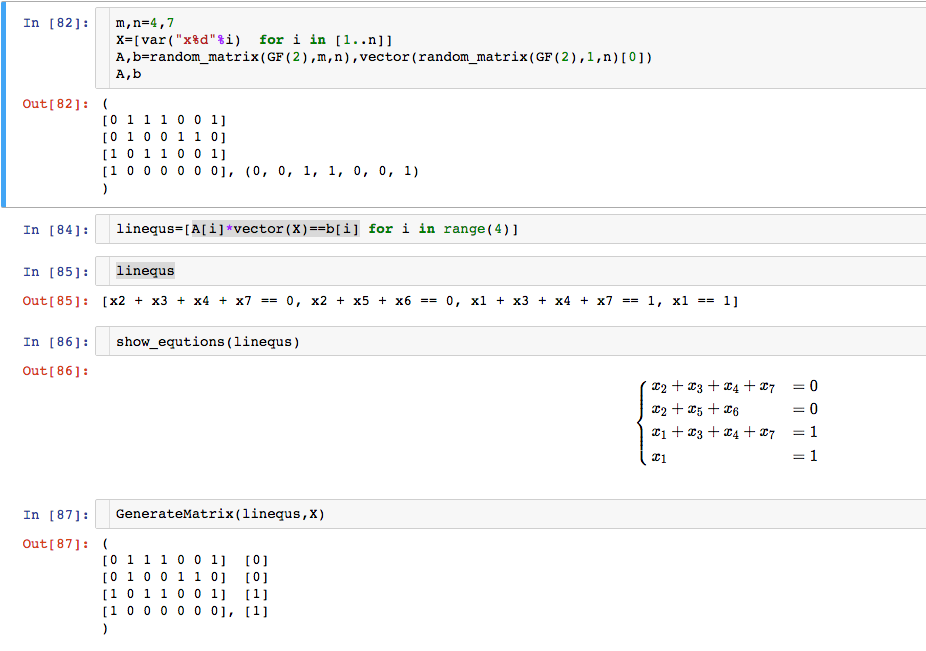Ask Your Question

# Revision history [back]

I give an answer for question 2. See the following :The definition of GenerateMatrix is:

def GenerateMatrix(equsys, vars):
A=matrix([[equ.lhs().coefficient(v) for v in vars] for equ in equsys])
b=matrix([[equ.rhs()] for equ in equsys])
return (A,b)